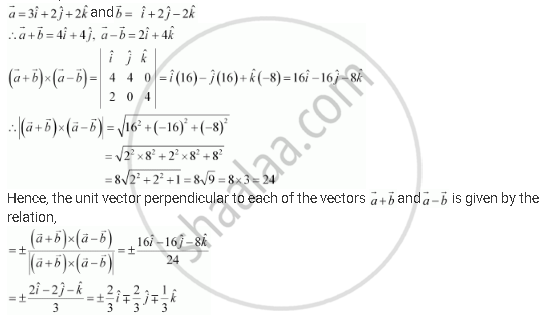Share

# Find a Unit Vector Perpendicular to Each of the Vector Veca + Vecb and Veca - Vecb, Where Veca = 3hati + 2hatj + 2hatk and Vecb = Hati + 2hatj - 2hatk. - CBSE (Science) Class 12 - Mathematics

ConceptProduct of Two Vectors Vector (Or Cross) Product of Two Vectors

#### Question

Find a unit vector perpendicular to each of the vector  veca  + vecb and veca - vecb, where veca = 3hati + 2hatj + 2hatk and vecb = hati + 2hatj  - 2hatk.

#### Solution

We have,Is there an error in this question or solution?

#### Video TutorialsVIEW ALL 

Solution Find a Unit Vector Perpendicular to Each of the Vector Veca + Vecb and Veca - Vecb, Where Veca = 3hati + 2hatj + 2hatk and Vecb = Hati + 2hatj - 2hatk. Concept: Product of Two Vectors - Vector (Or Cross) Product of Two Vectors.
S